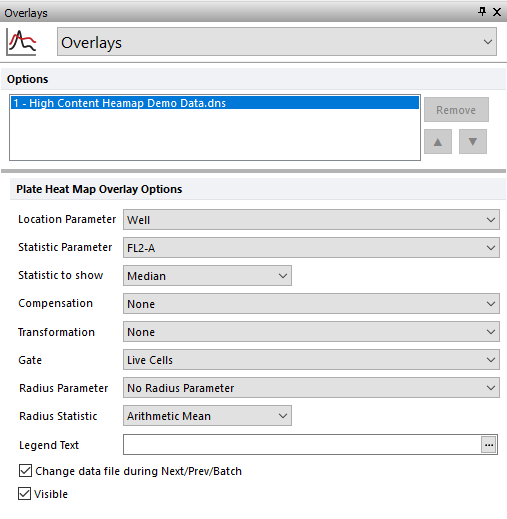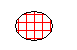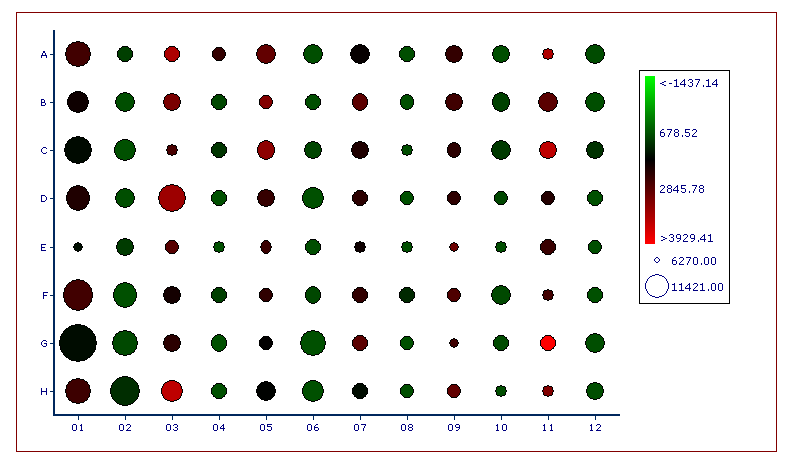﻿ Choosing Parameters and Statistics

# Choosing Parameters and Statistics

To select the parameter and statistic to display on the Plate Heat Map, use one of the following methods:

Select the Plate Heat Map and use the Format tabPlot OptionsOverlays command (Figure 5.84), and choose from the drop-down menus under Plate Heat Map Overlay Options.Figure 5.82 - Plate Heat Map Overlays Command from Ribbon

Right-click on the Plate Heat Map, select Format from the pop-up menu, and choose Overlays from the dropdown (Figure 5.85).

Click and hold down the mouse button over the y-axis label and choose an available parameter. Set the statistic to show using one of the above two methods.Figure 5.83 Plate Heat Map - Setting the Statistic Parameter and Statistic to Show

The data that is displayed is a combination of the Statistic parameter and the Statistic to show (e.g., Mean, Standard Deviation, CV, Median, # of events, 75%-ile) properties. FCS Express calculates the average of the statistic chosen across all of the wells in the microtiter plate. Then, a color is displayed for each well according to the color scheme property. The usual procedure is to assign the color by comparing the value of the statistic in each well to either:

The mean ± SD of the entire plate.

A predetermined threshold.

Choose the parameter from the Statistic parameter drop-down list. The list will be populated by parameters that were measured or calculated by your instrument. Select the Statistic to show from the predefined statistics in the corresponding drop-down list.

Available Statistics to Show:

 Min (or Max) value The Min (or Max) value is calculated for each well # of Event The number of events is calculated for each well % of parent For each well, the percentage of the Heatmap overlay gate is calculated using the parent gate as reference. This calculation is done on a per well bases. i.e. if Gate 2 is applied to the current Heatmap overlay and Gate 1 is the parent of Gate 2, the % of parent for each well will be calculated as the number of events in Gate 2 in a that well, divided by the number of events of Gate 1 in that same well, multiplied by 100. Note: in the previous version of FCS Express, the calculation was not performed on a per well bases, but on the whole plate instead. i.e. if Gate 2 was applied to the current Heatmap overlay, the % of parent was calculated as the number of events in Gate 2 in a given well, divided by the number of events displayed on the Heatmap (i.e. the total number of events in Gate 2), multiplied by 100. % of grandparent Same as above but the grandparent gate is used as reference. % of great grandparent Same as above but the great grandparent gate is used as reference. % of all cells Same as above but the total number of events in the well is used as reference. Median (or Geometric Mean, or Arithmetic Mean) The Median (or Geometric Mean, or Arithmetic Mean) for the parameter selected in Parameter Statistics drop-dow menu is calculated for each well. CV (or rCV) The Coefficient of Variation (or the robust Coefficient of Variation) for the parameter selected in Parameter Statistics drop-dow menu is calculated for each well. 5%-ile (or, 25%-ile, 75%-ile, 95%-ile) The selected 5th (or 25th, 75th, 95th) percentile for the parameter selected in Parameter Statistics drop-dow menu is calculated for each well. Concentration The concentration in each well is calculated based on the Concentration tool. Standard Deviation The Standard Deviation for the parameter selected in Parameter Statistics drop-dow menu is calculated for each well. BD-rSD (or BD-rCV) The BD robust Standard Deviation(or the BD robust Coefficient of Variation) for the parameter selected in Parameter Statistics drop-dow menu is calculated for each well.

 Please note: If a Statistic to Show for a well cannot be calculated, for instance, the Geometric Mean of a negative value, the well will appear                                                     "empty" with vertical and horizontal hatch marks as in the screen shot to the right. Choosing a different Statistic to Show may be appropriate.A Plate Heat Map can have a Gate set similar to any other plot in FCS Express. For example, you can draw a gate on a dot plot displaying the data and then drag the gate onto the plate heat map. You can also set the gate using the drop-down list in the Formatting window pictured above.